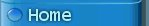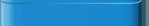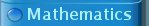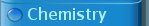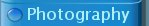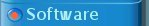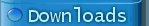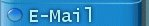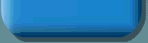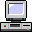### A tool for simulating linear electronic networks

In the past, I did quite some electronic engineering with audio circuits. I designed filters, amplifiers, equalizers etc. Usually, these circuits were based on 'linear' components and the design was done on the basis of idealized linear components, such as resistors, capacitors, opamps.

There are quite some software packages for simulating electronic circuits, but these are quite complicated and usually contain a database of many real-life components and are quite 'heavy' on a small computer system. What is missing is a simple program, which allows one to draw a simple scheme with some idealized components and getting a frequency and phase plot of the scheme over a specified range. This is where this program tries to fill a gap.

The networks program builds up a system of linear equations in the frequency domain (in fact, the Laplace domain) and solves this set of equations for each node in the scheme. The solution is obtained as a rational transfer function of the frequency (better: Laplace complex frequency s), with the node voltage as function of input current or input voltage. When this function is available, then it is easy to obtain an amplitude and phase plot as function of frequency.